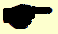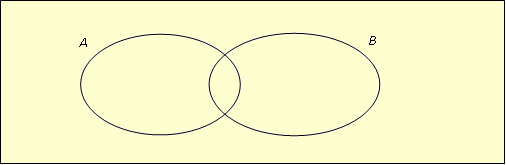# Mock exam paper B P1 Ordinary Level

 Name Class Teacher SchoolPRE – JUNIOR CERTIFICATE EXAMINATION, 2012

MATHEMATICS – ORDINARY LEVEL – Paper 1 (300 marks)

Time: 2 hours

Attempt ALL questions. Each question carries 50 marks.

Answers and supporting work should be written in the boxes provided.

Extra paper and graph paper can be obtained from the Superintendent, if needed.

The symbol indicates that supporting work must be shown to obtain full marks.

Make and model of calculator used

 Question Mark Adv. Exam. 1 2 3 4 5 6 Total Grade

For Superintendent / Examiner use only:

1. (a) and

Fill in the elements of A and B onto the following Venn diagram.(b) U is the universal set.

B

A

.7

. 9

. 2

1.

.3

6.

5.

.10

8.

.4

.11

.12

C

(i) List the elements of

(ii) List the elements of C’, the complement of the set C.

(iii) List the elements of .

(iv) Write down #C

(c) T is the set of natural numbers from 1 to 36, inclusive

(i) List the elements of T that are multiples of 4.

(ii) List the elements of T that are multiples of 6.

(iii)Write down the lowest common multiple of 4 and 6.

(iv)Express 30 as a product of its prime factors.

2. (a) If 5 litres of paint costs €7.25, find the cost of 9 litres.

(ii) By rounding each of these numbers to the nearest whole number, estimate the value of

(iii) Using a calculator, or otherwise, find the exact value of

(c) Using a calculator, or otherwise, find the exact value of

(i)

(ii)

(ii) Using a calculator, or otherwise, evaluate

3. (a) Kate bought two calculators at €12.99 each and four pens at €1.05 each. How much

change did she get if she paid with a €50 note?

(b) (i) Mary’s gross annual wage is €32,000. Her annual tax credit is €2,695 and she

pays income tax at the rate of 20%.What is Mary’s annual take home pay?

 Gross Pay €32,000 Tax @ 20% Tax Credit €2,695 Tax Due Take-home pay

(ii) A person bought a motorbike for €1,750 and sold it for €2,380. Calculate his profit as a

percentage of ...